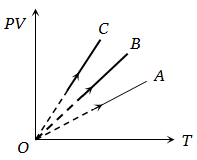NEET Physics Kinetic Theory of Gases Questions Solved

PV versus T graph of equal masses of ${\mathrm{H}}_{2}$$\mathrm{He}$ and ${\mathrm{O}}_{2}$ is shown in fig. Choose the correct alternative1. C corresponds to ${\mathrm{H}}_{2}$, B to $\mathrm{He}$ and A to ${\mathrm{O}}_{2}$

2. A corresponds to $\mathrm{He}$, B to ${\mathrm{H}}_{2}$ and C to ${\mathrm{O}}_{2}$

3. A corresponds to $\mathrm{He}$, B to ${\mathrm{O}}_{2}$ and C to ${\mathrm{H}}_{2}$

4. A corresponds to ${\mathrm{O}}_{2}$, B to ${\mathrm{H}}_{2}$ and C to ${\mathrm{H}}_{2}$

Explanation is a part of a Paid Course. To view Explanation Please buy the course.

Difficulty Level: